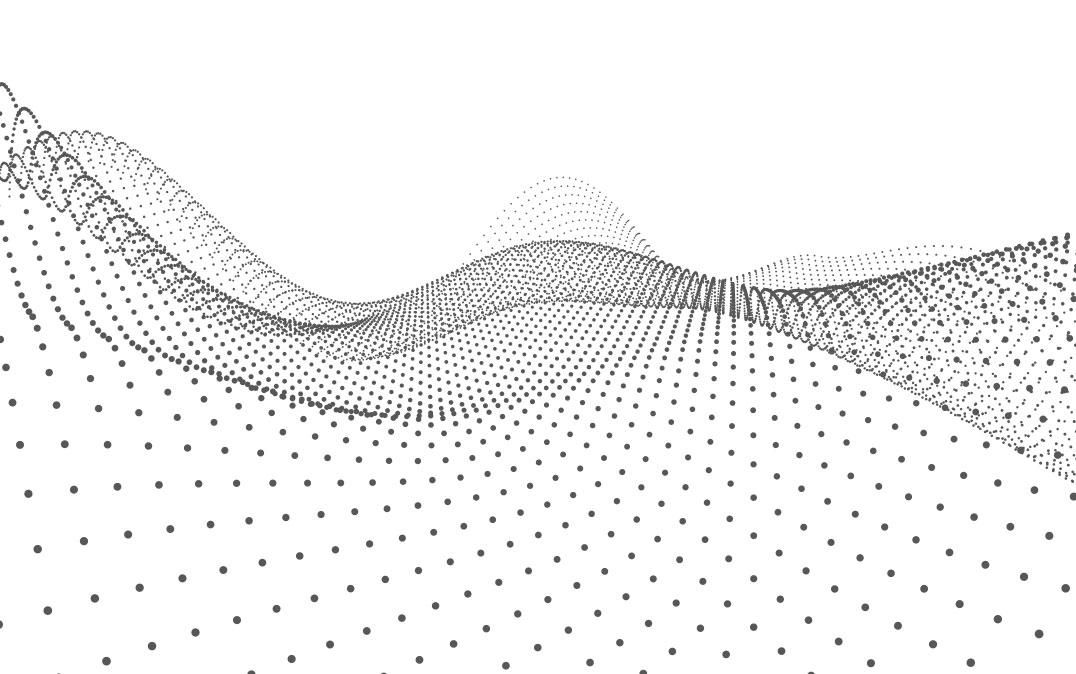## Research NewsImage by Kryshtofor Volodymyr/Shutterstock

Researchers at the University of Tsukuba employ a modified mathematical technique to make complicated nonlinear equations more tractable, which can lead to more accurate control systems for mechanical motions

Tsukuba, Japan—Researchers at the University of Tsukuba created a new method for deriving approximate linear equations to complicated nonlinear problems. Using simulation results, they show that the model derived using their proposed pseudo-linearization approach yields responses that are closer to those of the well-known alternative method. This work can help scientists and engineers predict and more precisely apply feedback control over mechanical systems that are described with nonlinear equations.

Mathematicians often separate equations into categories depending on how complicated they are to solve. Linear equations, in which variables would appear as a straight line when shown on a graph, are easier to deal with compared to nonlinear problems. However, as the physicist Stanislaw Ulam once said, "using a term like 'nonlinear science' is like referring to the bulk of zoology as the study of 'non-elephant animals." That is, the world we inhabit is complicated, and variables often depend on each other in convoluted ways. These relationships can lead to feedback loops or even chaotic instabilities, making predictions much more difficult. Thus, being limited to only linear equations would hinder scientists from modelling a great deal of important phenomena, including mechanical systems they want to control.

Unfortunately, a large portion of the mathematics that has been developed only works for linear equations. Having the ability to convert nonlinear dynamical systems into corresponding approximate linear versions would therefore be of great value. Now, researchers from the Department of Intelligent and Mechanical Interaction Systems at the University of Tsukuba have defined the concept of an 'equilibrium space,' which is a theoretical representation of all possible equilibria to the problem for which the system would be in equilibrium. The researchers consider their work to be a bridge between the abstract mathematics of nonlinear dynamical systems with an infinite number of equilibria and the real world of system control problems. "Although the basic concept has been proposed by scholars before, our definition of an abstract 'equilibrium space' brings it nearer to applications in engineering," says senior author Professor Triet NguyenŌĆæVan. Afterwards, pseudo-linearization can be performed, in which approximate linear equations could be calculated which have the same equilibrium states as the original problem.

To demonstrate the value of their method, the researchers used a simulation of a gyroscope that is free to rotate on gimbals about all three axes. Finding the steady-state behavior based on a given input torque was shown to be more accurate using their approach.

These findings can be applied to the design of nonlinear control systems in many situations. Some of these applications include keeping machines with many degrees of freedom from becoming unstable, thus improving performance and safety.

###
The authors have no relevant financial or non-financial interests to disclose.

### Original Paper

The work is published in Scientific Reports as "Equilibrium space and a pseudo linearization of nonlinear systems" at DOI: 10.1038/s41598-022-25616-1

### Correspondence

Assistant Professor NGUYEN Van Triet
Faculty of Engineering, Information and Systems, University of Tsukuba